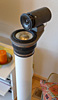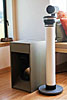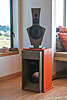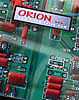----- Experience in your own room the magical nature of stereo sound -----

 Basics Issues in speaker design Dipole models Active filters Amplifiers etc Microphone FAQ's Loudspeakers Crossovers Room acoustics Stereo Recording and Rendering Audio production Conclusions

 Projects Your own desig LXmini LXmini+2 LXstudio LX521.4 LX521 reference ORION challenge ORION-3.4 PLUTO-2.1 WATSON-SEL PLUTO+ subwoofer THOR subwoofer PHOENIX dipole speaker Three-Box active system (1978) Reference earphones Surround sound Loudspeaker & Room

 Resources Sound recordings Links Other designs My current setup About me Site map

 ------------------ Digital Photo Processes

 ------------------ The Sea Ranch

 ------------------ My Daughter the Jeweler

# Issues in loudspeaker design - 3

| Page 1 | Page 2 | Page 3 | Page 4 | Page 5 | Page 6 | Page 7 |

O - Unreasonably high distortion in midrange drivers?

P - Estimate of Le(x) induced distortion at high frequencies

Q - Estimate of Le(i) induced distortion at high frequencies

R - Recommended study material about distortion in loudspeaker drivers

----------------------------------------------------------------------------------------------------------------------------------

## O - Unreasonably high distortion in midrange drivers?

The nonlinear relationship between SPL, excitation frequency and harmonic distortion products was investigated for three small midrange drivers as part of a search for an eventual replacement of the Peerless HDS 134, 850488, 5.25" driver that is used for PLUTO. Typically distortion increases with SPL or cone displacement. For constant SPL the displacement decreases as 1/f2 with increasing frequency and it could be expected that distortion decreases correspondingly.

In a linear system the output 'y' is proportional to the input 'x' as in
y = a1 x
In a nonlinear system the relationship between input and output is often of the form
y = a0 + a1 x + a2 x2 + a3 x3 + .....+ an xn    which is a power series expanded around x = 0.
If the input is a sinusoidal voltage
x = A sin(
wt), then
x2 = A2 [1 - 0.5 cos(2
wt)]
x3 = A3 [0.75 sin(
wt) - 0.25 sin(3wt)]
x4 = A4 [0.375 - 0.5 cos(2
wt) + 0.125 cos(4wt)]
and so on for higher powers of x. Thus harmonics n
w are generated and dc terms.w will also decrease by dA (-1 dB), but the second harmonic amplitude will decrease by dA2 (-2 dB), the third harmonic amplitude by dA3 (-3 dB), the fourth by dA4 (-4 dB), and so on, at least to a first order approximation.

The sound output from the unbaffled driver was measured with the microphone in the center of the plane that touches the driver's roll surround. Drive voltages are listed for 125 dB SPL. The amplitude of the harmonics is plotted relative to the fundamental. The fundamental amplitude was changed in 5 dB increments from 105 dB to 125 dB SPL. The dashed lines x show the relative change in cone excursion with SPL and frequency. Driver A is the Peerless 850488, the other two drivers were samples under consideration.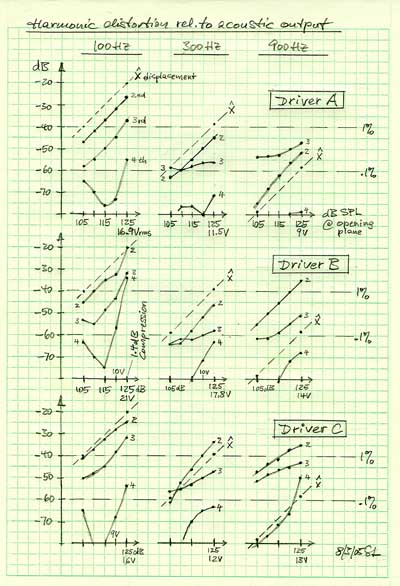Driver A 100 Hz - The relative amplitude change of the 2nd harmonic behaves exactly as expected. The 3rd harmonic starts out with the right slope for high SPL but then decreases more slowly. The 4th behaves strange at low levels. 300 Hz - Excursion x has decreased by 19 dB. The 2nd harmonic follows but not the others. 900 Hz - Excursion x has decreased by 38 dB. The distortion products do not drop correspondingly. Driver B 100 Hz - Slightly higher distortion than driver A. Serious misbehavior above 120 dB SPL. 300 Hz - Slightly lower distortion than driver A and similar behavior. 900 Hz - Large amount of 2nd harmonic. Again all distortion products are much higher than expected from the reduction in excursion. Driver C 100 Hz - Worse than drivers A and B.  300 Hz - The 2nd and 3rd harmonics have dropped less than in the other drivers. 900 Hz - High levels of distortion.

The dominant nonlinear parameters in a driver are the force factor Bl(x), the stiffness of suspension Kms(x), and the electrical inductance Le(x). The effect of Bl(x) and Kms(x) should decrease as 1/w2 with frequency, but since the inductance increases as wLe its effect should only decrease as 1/w and possibly overtake the effects of the other two non-linearities. At least that is what has been suggested to me. All these parameters can be measured with a Klippel Analyzer, at least for low frequencies. In the following I will estimate the Le(x) induced distortion based on measured Le(x) for drivers A and B.
Top

## P - Estimate of Le(x) induced distortion at high frequencies

The voice coil inductance changes with displacement x in a nonlinear fashion as seen is the graphs for Driver A and Driver B. The behavior for small excursions around  x = 0  can be approximated by the two expressions for Le below.LeA = 410 mH - 70 mH (x / 5 mm)2LeB = 305 mH + (70 mH / 7.5 mm) x

In general, Le(x) can be expanded as   Le(x) = L0 + L1 x + L2 x2 + ....  
where  L0A = 410
mH,   L1A = 0,   L2A = 2.8 mH/mm2
and  L0B = 305
mH,   L1B = 9.33 mH/mm

### 1 - Electrical impedance change due to Le(x)

With a current i flowing through the coil the reactive voltage across the inductance Le(x) becomes both a function of i and of resulting displacement x

vLe = Le di/dt + i (dLe/dx) (dx/dt)    

The displacement x follows from the relationship between applied force F = Bli and cone acceleration a in the mass controlled region of driver operation far above fs

F = Bli = Mms a    

For sinusoidal displacement  x = X sin(wt)
the velocity is   dx/dt =
w cos(wt) = w x    
and the acceleration is   a = d2x/dt2 = -
w2 x
thus  Bli = Mms
w2 x
and   x = Bli / (
w2 Mms) = K i    
where   K = Bl / (
w2 Mms)

 The current is  i = v1 / Z     and for sinusoidal voltage   v1 = Vs sin(wt)   the current becomes   i = (Vs/Z) sin(wt)  and thus di/dt = w (Vs/Z) cos(wt) = w i     Z = sqrt [ (Re)2 + (wL0)2 ]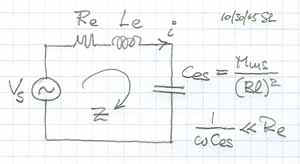Finally from differentiating  above  dLe/dx = L1 + 2 L2 x    

Inserting [4, 5, 7, 9] into  yields the voltage across the inductance

vLe = i wL0 + i2 K wL1 + i3 2 K2 wL2    

Where
i = v1 / Z = (Vs/Z) sin(
wt)
i2 = (Vs/Z)2 [1 - 0.5 cos(2
wt)]
i3 = (Vs/Z)3 [0.75 sin(
wt) - 0.25 sin(3wt)]

So approximately   vLe = i wL0 - 0.5 (Vs/Z)2 K wL1 cos(2wt) - 0.5 (Vs/Z)3 K2 wL2 sin(3wt)

 With a 2nd harmonic generator   v2 = 0.5 (Vs/Z)2 K wL1     and a 3rd harmonic generator    v3 = 0.5 (Vs/Z)3 K2 wL2    the relative distortion  becomes  d2 = v2/Vs = 0.5 (wL1/Z) (K Vs/Z)        and    d3 = v3/Vs = 0.5 (wL2/Z) (K Vs/Z)2Expressed in dB the distortion is   D2 = 20 log(d2)   and   D3 = 20 log(d3)

Numerical example 1 for f = 900 Hz

 Quantity Driver A Driver B Units Vs 9 14 Volt Z = sqrt [ (Re)2 + (wL0)2 ] 6.15 6.24 ohm Vs/Z 1.46 2.24 A K = Bl / (w2 Mms) 21.2e-3 12.5e-3 mm/A (wL1/Z) ---- 8.46e-3 1/mm (wL2/Z) 2.57e-3 ---- 1/mm2 D2 ---- -78 dB D2 measured -52 -35 dB D3 -118 ---- dB D3 measured -47 -51 dB Difference 71 43 dB

Clearly there is a huge difference between the distortion estimates and the measured values. The questions is why? The mechanical displacements are extremely small and in the order of 30 micron. The displacements that produce the measured distortion are  less than 1 mm. Which nonlinear mechanism is causing the distortion? The currents, magnetic fields, forces and accelerations are the same as at low frequencies for constant SPL, only the excursions are drastically reduced.

### 2 - Force generated due to Le(x)

There is a second effect due to Le(x) that must be considered which is the reluctance force FL. This force has its useful application in electro-magnetic transducers like door bells and early headphones. Here it is an undesirable remnant. Its magnitude is derived from the energy that is stored in the magnetic field of the voice coil.

W = 0.5 i2 Le(x)
dW/dx = FL = 0.5 i2 dLe(x) / dx

This force will generate 2nd harmonic distortion with an amplitude d2 relative to F = Bli

d2 = FL / F = 0.5 i [dLe(x) / dx] / (Bl)    

Numerical example 2 for f = 900 Hz

 Quantity Driver A Driver B Units dLe(x) / dx 0 9.33 mH/mm i 1.46 2.24 A Bl 6.9 4.5 N/A D2 - infinity -53 dB D2 measured -52 -35 dB Difference infinity 18 dB

For Driver A the gradient of Le(x) is zero and thus this type of distortion is zero. For Driver B the calculated distortion is still by an order of magnitude lower than the measured value. Thus I draw the conclusion that in these two cases the reluctance force is not the dominant factor.

Estimates 1 and 2 do not mean, though, that Le(x) is insignificant for distortion at high frequencies. They only mean that for a high frequency excitation signal, with its very small voice coil displacement x, there is no significant amount of harmonic distortion generated. But, if a low frequency signal is present together with the high frequency signal, then the large low frequency voice coil displacements can cause large amounts of intermodulation distortion products near the high frequency signal due to the large low frequency variation of Le(x).
Top

## Q - Estimate of Le(i) induced distortion at high frequencies

The voice coil inductance changes also as a function of the current flowing through it. The magnetic field strength H(i) that is generated by the voice coil current produces nonlinear increases and decreases mH(i) of the static magnetic bias flux density B that is generated by the permanent magnet. The permeability m of the magnetic circuit changes nonlinearly and with hysteresis losses.

The relationship between magnetic flux F and inductance is  F = Le(i) i   where    F = [ B + mH(i) ] A

A time change in flux generates a voltage   v = - dF / dt = - i (dLe(i)/di) (di/dt)

Thus the voltage across the voice coil can be described by

vLe = Le di/dt - i (dLe(i)/di) (di/dt)    

where   di/dt = wi   and   i = Vs/Z   and thus

vLe = wLe - i2 w dLe(i)/di    which contains a 2nd harmonic distortion generator   (Vs/Z)2 w dLe(i)/di

Similar to the model for  above:

d2 = (Vs/Z2) w dLe(i)/di        due to flux modulation

The value of dLe(i)/di is not known to me for drivers A and B. I have seen data for a driver where the inductance changed in the order of 3% per ampere without shorting rings and 2%/A with shorting rings.
For the calculation below I have used a value of 3%/A.

Numerical example 3 for f = 900 Hz

 Quantity Driver A Driver B Units Le 410 305 mH dLe(i) / di 12 9 mH/A Z 6.15 6.24 ohm D2 - 36 -35 dB D2 measured -52 -35 dB Difference -16 0 dB

It appears that flux modulation is the dominant mechanism for harmonic distortion at high frequencies in these drivers. Driver A actually has shorting rings and Le(i) could not have changed by more than 0.5%/A in order to match the calculated value with the measurement. That may indicate a really good choice of materials and design.

In summary, the measured values do not seem unreasonable, but there is certainly room for improvement of drivers B and C.
Top

-----------------------------------------------------------------------

## R - Recommended study material about distortion in loudspeaker drivers

1 - Wolfgang Klippel, "Loudspeaker Nonlinearities - Causes, Parameters, Symptoms", 119th AES Convention, New York, October 2005, Preprint 6584

2 - The following two cutouts are from a new and comprehensive poster "Loudspeaker Nonlinearities - Causes, Parameters, Symptoms" by Klippel at CES 2006The two stimulus measurement method allows to see and separate out the effects of the dominant driver nonlinearities by measuring distortion in sound pressure, distortion of the voice coil current and dc-displacement.

The table below indicates in which form the symptoms of the nonlinearities show up in the measurements.3 - "The power of Loudspeaker Models", W. Klippel, Richard Small, David Clark, Jürgen Ringlstetter, Andrew Bright
Panel Workshop, AES SAN FRANCISCO 2004, Transparencies,  PDF

4 - Klippel, "Interactive Listening Test"

5 - Mark Dodd, Wolfgang Klippel, Jack Oclee-Brown, "Voice Coil Impedance as a Function of Frequency and Displacement", 117th AES Convention, San Francisco, October 2004, Preprint 6178
(The effects of impedance variation with current and magnetic hysteresis are not considered in this paper.)

Other nonlinear and linear distortion measurements and procedures can be found on the following pages:

Distortion test of drivers

Woofer measurements

Audibility of allpass crossover phase distortion

Diffraction from baffle edges

-------------------------------------------------------------------

| Page 1 | Page 2 | Page 3 | Page 4 | Page 5 | Page 6 | Page 7 |What you hear is not the air pressure variation in itself  but what has drawn your attention in the streams of superimposed air pressure variations  at your eardrums An acoustic event has dimensions of Time, Tone, Loudness and Space Have they been recorded and rendered sensibly? ___________________________________________________________ Last revised: 01/18/2023   -  © 1999-2019 LINKWITZ LAB, All Rights Reserved